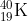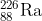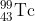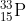×
Get Full Access to Chemistry: A Molecular Approach - 3 Edition - Chapter 2 - Problem 54e
Get Full Access to Chemistry: A Molecular Approach - 3 Edition - Chapter 2 - Problem 54e

×

# Solved: Determine the number of protons and the number ofISBN: 9780321809247 1

## Solution for problem 54E Chapter 2

Chemistry: A Molecular Approach | 3rd Edition

• Textbook Solutions
• 2901 Step-by-step solutions solved by professors and subject experts
• Get 24/7 help from StudySoup virtual teaching assistantsChemistry: A Molecular Approach | 3rd Edition

4 5 1 252 Reviews
26
2
Problem 54E

Determine the number of protons and the number of neutrons in each isotope.

a.b.c.d.Step-by-Step Solution:

Solution : First let’s see what is molecular formula and structural formula and then we will write the formulas for the given compounds. Step 1 : Molecular formula is a chemical formula that gives the total number of atoms of each element in each molecule of a substance For Example : C H2s6he molecular formula of ethane. 1 molecule of ethane contains 2 atoms of Carbon and 6 hydrogen (H) atoms. Structural formula represents the order in which the atoms in a molecule are connected that is it shows the arrangement within the molecule of a toms a nd bonds. It is a graphic structure showing the ar rangement of atoms. For Example : The structural formula for ethane (C 26 is : The black colored atom is Carbon(C), red colored atom is Oxygen(O) and white colored atom is Hydrogen(H), others are mentioned in the compounds given.

Step 2 of 2

## Discover and learn what students are asking

Calculus: Early Transcendental Functions : Linear Models and Rates of Change
?In Exercises 5-10, plot the pair of points and find the slope of the line passing through them. (4,6), (4,1)

Statistics: Informed Decisions Using Data : An Overview of Nonparametric Statistics
?List the disadvantages of using nonparametric statistical procedures

Unlock Textbook Solution